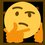# Derivation of the Elastic Collision Formula

In the scenario of an one-dimensional elastic collision between two objects, $1$ and $2$, their final velocities, $v_1$ and $v_2$ can be found with the following formula knowing their individual masses, $m_1$ and $m_2$, and their initial velocities, $u_1$ and $u_2$.

Derivation:

In an elastic collision, momentum is conserved:

\begin{aligned} \sum p_f&=\sum p_i\\ m_1v_1+m_2v_2&=m_1u_1+m_2u_2\\ m_1v_1-m_1u_1&=m_2u_2-m_2v_2\\ \textcolor{#3D99F6}{m_1(v_1-u_1)}&=\textcolor{#3D99F6}{m_2(u_2-v_2)} \end{aligned}

In an elastic collision, kinetic energy is conserved:

\begin{aligned} \sum K_f&=\sum K_i\\ \frac12m_1v_1^2+\frac12m_2v_2^2&=\frac12m_1u_1^2+\frac12m_2u_2^2\\ m_1v_1^2+m_2v_2^2&=m_1u_1^2+m_2u_2^2\\ m_1v_1^2-m_1u_1^2&=m_2u_2^2-m_2v_2^2\\ m_1(v_1^2-u_1^2)&=m_2(u_2^2-v_2^2)\\ \textcolor{#3D99F6}{m_1(v_1-u_1)}(v_1+u_1)&=m_2(u_2-v_2)(u_2+v_2)\\ \textcolor{#3D99F6}{m_2(u_2-v_2)}(v_1+u_1)&=m_2(u_2-v_2)(u_2+v_2)\\ v_1+u_1&=v_2+u_2\\ \textcolor{#20A900}{v_2}&=\textcolor{#20A900}{u_1+v_1-u_2} \end{aligned}

\begin{aligned} \sum p_f&=\sum p_i\\ m_1v_1+m_2\textcolor{#20A900}{v_2}&=m_1u_1+m_2u_2\\ m_1v_1+m_2(\textcolor{#20A900}{u_1+v_1-u_2})&=m_1u_1+m_2u_2\\ m_1v_1+m_2u_1+m_2v_1-m_2u_2&=m_1u_1+m_2u_2\\ m_1v_1+m_2v_1&=m_1u_1+2m_2u_2-m_2u_1\\ (m_1+m_2)v_1&=(m_1-m_2)u_1+2m_2u_2\\ \textcolor{#D61F06}{v_1}&=\textcolor{#D61F06}{\left(\frac{m_1-m_2}{m_1+m_2}\right)u_1+\left(\frac{2m_2}{m_1+m_2}\right)u_2} \end{aligned}

\begin{aligned} v_2&=u_1+\textcolor{#D61F06}{v_1}-u_2\\ v_2&=u_1+\textcolor{#D61F06}{\left(\frac{m_1-m_2}{m_1+m_2}\right)u_1+\left(\frac{2m_2}{m_1+m_2}\right)u_2}-u_2\\ v_2&=\left(1+\frac{m_1-m_2}{m_1+m_2}\right)u_1+\left(\frac{2m_2}{m_1+m_2}-1\right)u_2\\ v_2&=\left(\frac{m_1+m_2}{m_1+m_2}+\frac{m_1-m_2}{m_1+m_2}\right)u_1+\left(\frac{2m_2}{m_1+m_2}-\frac{m_1+m_2}{m_1+m_2}\right)u_2\\ v_2&=\left(\frac{2m_1}{m_1+m_2}\right)u_1+\left(\frac{m_2-m_1}{m_1+m_2}\right)u_2 \end{aligned}

\therefore \boxed{ \begin{aligned} v_1&=\left(\frac{m_1-m_2}{m_1+m_2}\right)u_1+\left(\frac{2m_2}{m_1+m_2}\right)u_2\\ v_2&=\left(\frac{2m_1}{m_1+m_2}\right)u_1+\left(\frac{m_2-m_1}{m_1+m_2}\right)u_2 \end{aligned} }

or, equivalently:

$\boxed{ \def\arraystretch{1.5} \begin{bmatrix} v_1\\v_2 \end{bmatrix}= \frac1{m_1+m_2}\begin{bmatrix} m_1-m_2&2m_2\\ 2m_1&m_2-m_1 \end{bmatrix} \begin{bmatrix} u_1\\u_2 \end{bmatrix} }$Note by Gandoff Tan
1 year, 7 months ago

This discussion board is a place to discuss our Daily Challenges and the math and science related to those challenges. Explanations are more than just a solution — they should explain the steps and thinking strategies that you used to obtain the solution. Comments should further the discussion of math and science.

When posting on Brilliant:

• Use the emojis to react to an explanation, whether you're congratulating a job well done , or just really confused .
• Ask specific questions about the challenge or the steps in somebody's explanation. Well-posed questions can add a lot to the discussion, but posting "I don't understand!" doesn't help anyone.
• Try to contribute something new to the discussion, whether it is an extension, generalization or other idea related to the challenge.

MarkdownAppears as
*italics* or _italics_ italics
**bold** or __bold__ bold
- bulleted- list
• bulleted
• list
1. numbered2. list
1. numbered
2. list
Note: you must add a full line of space before and after lists for them to show up correctly
paragraph 1paragraph 2

paragraph 1

paragraph 2

[example link](https://brilliant.org)example link
> This is a quote
This is a quote
    # I indented these lines
# 4 spaces, and now they show
# up as a code block.

print "hello world"
# I indented these lines
# 4 spaces, and now they show
# up as a code block.

print "hello world"
MathAppears as
Remember to wrap math in $$ ... $$ or $ ... $ to ensure proper formatting.
2 \times 3 $2 \times 3$
2^{34} $2^{34}$
a_{i-1} $a_{i-1}$
\frac{2}{3} $\frac{2}{3}$
\sqrt{2} $\sqrt{2}$
\sum_{i=1}^3 $\sum_{i=1}^3$
\sin \theta $\sin \theta$
\boxed{123} $\boxed{123}$